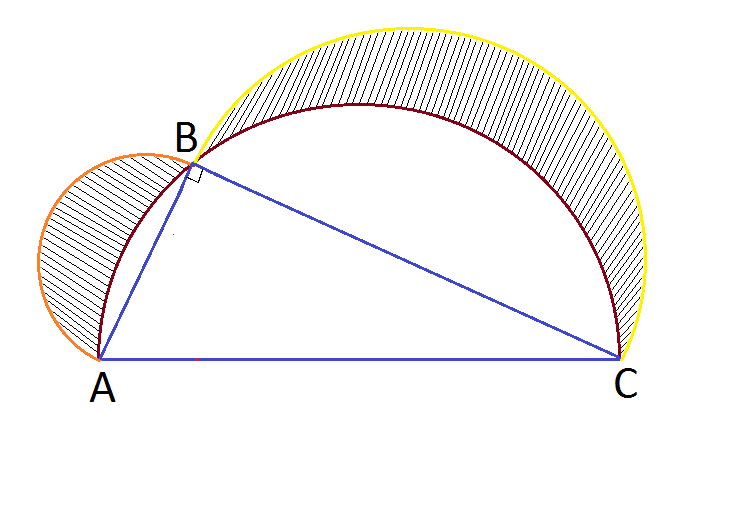# Semi Semi Circles

Geometry Level 1In the figure above, semicircles are constructed on the hypotenuse and legs of a right angle triangle. If $S_{1 }$, $S_{2 }$ are the area of shaded regions and $S$ is the area of right angled triangle, which of the following is true ?

×